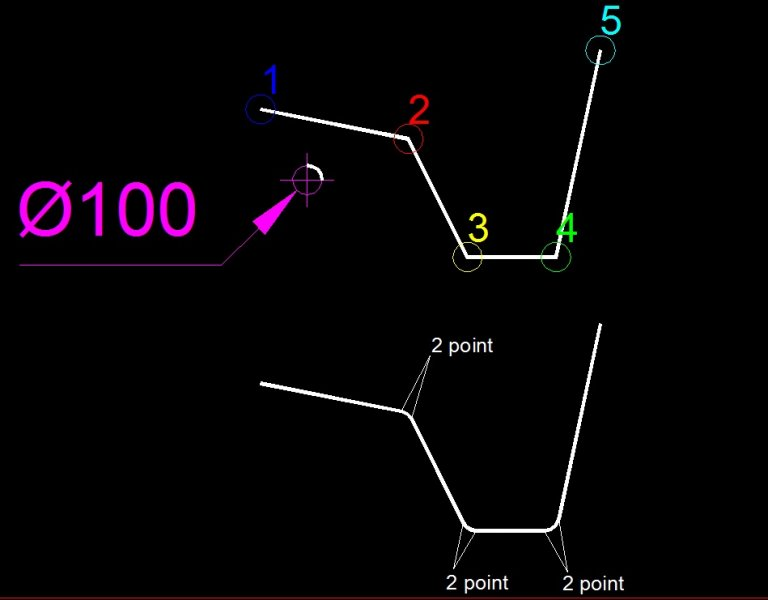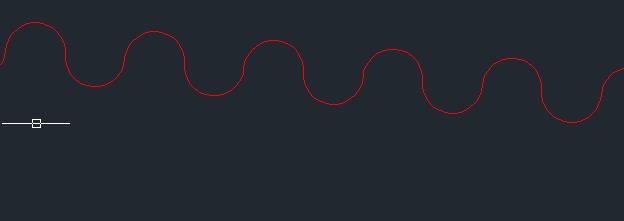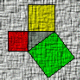# Polyline - bulge## Recommended PostsHello

I would like to create polylines not using the command, have data point (1,2,3,4,5) as the attachment sketch and diameter bending.

How to calculate the start and end points of the arc regardless of the direction and the correct value bulge##### Share on other sitesFirst calculate the position of the arc endpoints & center (based on the adjacent polyline segments and the required arc radius), and then the bulge for the arc segment is equal to the tangent of a quarter of the included angle.

Refer to my Bulge Conversion Functions for more information.

##### Share on other sitesHi, Thank you for interesting reading. It turns out that my problem that I have overcome the first calculations are ordinates in a coordinate system.I hope that soon wish to train.

##### Share on other sitesone more interesting link to ElpanovEvgeniy web site: Replacement of a linear segment of a polyline with an arc segment##### Share on other sitesI started by writing a function of calculating the angle between the vectors. Unfortunately, it seems to me that somewhere in my reasoning is wrong . Could you look at the function below.

```(defun wek (Pk1 Pk2 Pk3 / W1 W2 CosAlfa)
(setq W1 (list (- (car Pk2) (car Pk1)) (- (cadr Pk2) (cadr Pk1)))) ;wsp1
(setq W2 (list (- (car Pk3) (car Pk2)) (- (cadr Pk3) (cadr Pk2)))) ;wsp2
(setq CosAlfa (/ (+ (* (car W1)(car W2)) (* (cadr W1)(cadr W2)))
(*(sqrt (+ (* (car W1) (car W1)) (*(car W2) (car W2) )))
)
(RtD (atan (sqrt (- 1 (* CosAlfa CosAlfa))) CosAlfa))
)
; converts radians to degrees
(defun RtD (r) (* 180.0 (/ r pi)))
; converts degrees to radians
(defun DtR (d) (* pi (/ d 180.0)))```

##### Share on other sites1st question do you want one radius for all if so then search here the code for that is done. Also re angles its best to work in radians as this is the default for autocad but be aware zero is to the east and they are measured clock wise.

You can get the co-ords of a pline as a list so you would just take pt1 pt2, pt2 pt3 to get angles in lisp (angle pt1 pt2)

Here is a co-ord lisp example

```; pline co-ords example
; By Alan H
(defun getcoords (ent)
(vlax-safearray->list
(vlax-variant-value
(vlax-get-property
(vlax-ename->vla-object ent)
"Coordinates"
)
)
)
)

(defun co-ords2xy ()
; convert now to a list of xy as co-ords are x y x y x y if 3d x y z x y z
(setq len (length co-ords))
(setq numb (/ len 2)) ; even and odd check required
(setq I 0)
(repeat numb
(setq xy (list (nth i co-ords)(nth (+ I 1) co-ords) ))
; odd (setq xy (list (nth i co-ords)(nth (+ I 1) co-ords)(nth (+ I 2) co-ords) ))
(setq co-ordsxy (cons xy co-ordsxy))
(setq I (+ I 2))
)
)

; program starts here
(setq co-ords (getcoords (car (entsel "\nplease pick pline"))))
(co-ords2xy) ; list of 2d points making pline

(princ co-ordsxy)
```

something like this its a two point pick##### Share on other sitesjan_ek

I started by writing a function of calculating the angle between the vectors.

Jan,

Simply use the angle function.

```(defun ang3p (p1 p2 p3 / a)
(or 2pi (setq 2pi (* 2 pi)))
(abs (- (setq a (- (angle p2 p1)(angle p2 p3))) (* (fix (/ a pi)) 2pi)))
)
```

ymg

##### Share on other sitesmaybe this topic will be useful

Challenge - Find interior angles of a closed polyline

Convert GEOMCAL functions to LISP

Author: Gregory Cherevko

===========================

No liability for the use of these texts and the possible errors the authors did not carry.

functions are written in AutoLisp

All calculations are made by mathematical methods (vector algebra, analytic geometry) without using auxiliary AutoCAD entities and their analysis.

Global variables

Many library functions refer to two global variables

The variable \$ P000 has the value '(0.0 0.0 0.0)

\$ Dopusk has a value of 0.00001 - contains the error value is used for various comparisons (such as coordinates of points). He just needed to cope with the accumulated error in the calculations.

To initialize variables in the start of your program to insert the line

(Setq \$ P000 '(0.0 0.0 0.0)

\$ Dopusk 0.00001

)

Naming of variables, parameters

In the parameters of these functions are frequently used variable names.

P, P1, P2, P3 .... - Points

Pc - the central point of an arc or a circle

L, Lx, Ly, Lz - length

Wekt, Wekt1, Wekt2, WektX, WektY, WektZ, W, W1, W2-vector (the list of 3 numbers)

WNorm - normal vector. In terms of AutoCAD it comes to squeezing the direction vector, which all primitives stored under 210 associative code.

Ang - angle

R, R1, R2 - radius

================================================== ====================

Calculations of the arcs

1. Distance from a point in the plane of the arc to arc (1.zip)

2. Calculating the arc length (2.zip)

3. Calculation of the central angle of arc (3.zip)

4. Computation of the mid-point arc (4.zip)

5. Calculation of the center of the arc (the arc is defined by 2 points and the direction

tangent to the 1-th point) (5.zip)

Analysis functions belonging and intersection elements

6. Accessory points on the line, set point and the vector (15.zip)

7. Affiliation point interval (16.zip)

8. Affiliation point arc (all points in the same plane!) (17.zip)

9. Affiliation point arc (points in different planes!) (18.zip)

10. Affiliation arc arc (19.zip)

Function calculating the intersection of objects in space

11. The intersection of 2 circles given center and radius,

coplanar (10.zip)

12. The intersection of 2 circles given center and radius,

located in a given plane (11.zip)

13. Point of intersection of the line and plane (12.zip)

14. Point of intersection of the circle with the plane (13.zip)

15. Calculation of the intersection line of planes (14.zip)

16. Intersection of line and circle, lying in one plane (8.zip)

17. Intersection of line and circle, randomly located (9.zip)

General purpose functions

18. Vector product of vectors (20.zip)

19. Vector normal to the plane. The plane defined by 3 points (21.zip)

20. Calculation of the unit vector (unit vector) (22.zip)

21. Reduction of the vector to the length of 1000 (long vector) (23.zip)

22. Point lying on the point P1 at a distance L in the direction of the point P2 (24.zip)

23. Point lying on P1 at a distance L along the vector Wekt (25.zip)

24. Point, offset by a 2-m vectors (26.zip)

25. The condition of orthogonality (27.zip)

26. The angle between the vectors (29.zip)

27. The angle between the vectors in a clockwise direction from the normal (30.zip)

28. Vector angle with respect to this part of the normal (31.zip)

29. The nearest point from a given point of the segment (32.zip)

30. The distance from the point to the segment (33.zip)

31. Rotate the point around the axis (34.zip)

32. Projection of a point on the line (line set-point vector) (35.zip)

33. Projection of a point on the line (line given two points) (36.zip)

34. Projection of a point on the plane (37.zip)

35. The distance between the planes (38.zip)

Function of spatial geometry

36. Calculation of the point of contact from the point of the circle (6.zip)

37. The calculation of the points of contact to the 2-m circles (7.zip)

================================================== =======================

3d_lib.zip

Edited by VVA
##### Share on other sitesLook Jan, if you are trying to fillet polylines with circle at their vertices, try this code :

```(defun c:cirfilletpls ( / *error* vertlst bulglst *adoc* pea ucsf ss r i ent vertl hf ssent ss2l v1l1 v2l1 v1l2 v2l2 v1 v11 v2 v22 ip v3 )

(defun *error* ( msg )
(if pea (setvar 'peditaccept pea))
(if msg (prompt msg))
(princ)
)

(defun vertlst ( pl / k p vertl ) (vl-load-com)
(setq k -1.0)
(while (setq p (vlax-curve-getpointatparam pl (setq k (1+ k))))
(setq vertl (cons p vertl))
)
(reverse vertl)
)

(defun bulglst ( pl / k b bulgl ) (vl-load-com)
(setq k -1.0)
(while (not (eq (cdr (assoc 90 (entget pl))) (fix (setq k (1+ k)))))
(setq b (vla-getbulge (vlax-ename->vla-object pl) k))
(setq bulgl (cons b bulgl))
)
(reverse bulgl)
)

(setq pea (getvar 'peditaccept))
(if (eq (getvar 'worlducs) 0)
(progn
(command "_.UCS" "_W")
(setq ucsf t)
)
)
(prompt "\nSelect POLYLINE POLYGONS that are placed in WCS plane and are on unlocked layer(s)...")
(setq ss (ssget "_:L" (list '(0 . "*POLYLINE") '(-4 . "<or") '(70 . 0) '(70 . 1) '(70 . 128) '(70 . 129) '(-4 . "or>"))))
(while (not ss)
(prompt "\nEmpty sel.set... Please select POLYLINE POLYGONS that are placed in WCS plane and are on unlocked layer(s) again...")
(setq ss (ssget "_:L" (list '(0 . "*POLYLINE") '(-4 . "<or") '(70 . 0) '(70 . 1) '(70 . 128) '(70 . 129) '(-4 . "or>"))))
)
(acet-ss-zoom-extents ss)
(initget 7)
(setq r (getdist "\nPick or specify radius of cirfillet along vertices - must be smaller than half distance of smallest straight segment : "))
(repeat (setq i (sslength ss))
(setq ent (ssname ss (setq i (1- i))))
(if (eq (cdr (assoc 0 (entget ent))) "POLYLINE")
(progn
(setq hf t)
(command "_.CONVERTPOLY" "_L" ent)
(while (> (getvar 'cmdactive) 0) (command ""))
(entupd ent)
)
)
(if
(and
(vl-every '(lambda ( v ) (equal (caddr v) 0.0 1e-) (setq vertl (vertlst ent)))
(vl-every '(lambda ( b ) (equal b 0.0 1e-) (bulglst ent))
(< r (/ (car (vl-sort (mapcar '(lambda ( a b ) (distance a b)) vertl (cdr vertl)) '<)) 2.0))
)
(progn
(if (eq (logand (cdr (assoc 70 (entget ent))) 1) 1)
(progn
(command "_.EXPLODE" ent)
(while (> (getvar 'cmdactive) 0) (command ""))
(setq ssent (ssget "_P"))
(foreach v (reverse (cdr (reverse vertl)))
(setq ss2l (ssget "_C" v v))
(setq v1l1 (vlax-curve-getstartpoint (ssname ss2l 0)))
(setq v2l1 (vlax-curve-getendpoint (ssname ss2l 0)))
(setq v1l2 (vlax-curve-getstartpoint (ssname ss2l 1)))
(setq v2l2 (vlax-curve-getendpoint (ssname ss2l 1)))
(if (equal v v1l1 1e-
(progn
(entupd (cdr (assoc -1 (entmod (subst (cons 10 (polar v (angle v v2l1) r)) (assoc 10 (entget (ssname ss2l 0))) (entget (ssname ss2l 0)))))))
(setq v1l1 (polar v (angle v v2l1) r))
)
)
(if (equal v v2l1 1e-
(progn
(entupd (cdr (assoc -1 (entmod (subst (cons 11 (polar v (angle v v1l1) r)) (assoc 11 (entget (ssname ss2l 0))) (entget (ssname ss2l 0)))))))
(setq v2l1 (polar v (angle v v1l1) r))
)
)
(if (equal v v1l2 1e-
(progn
(entupd (cdr (assoc -1 (entmod (subst (cons 10 (polar v (angle v v2l2) r)) (assoc 10 (entget (ssname ss2l 1))) (entget (ssname ss2l 1)))))))
(setq v1l2 (polar v (angle v v2l2) r))
)
)
(if (equal v v2l2 1e-
(progn
(entupd (cdr (assoc -1 (entmod (subst (cons 11 (polar v (angle v v1l2) r)) (assoc 11 (entget (ssname ss2l 1))) (entget (ssname ss2l 1)))))))
(setq v2l2 (polar v (angle v v1l2) r))
)
)
(if (< (distance v v1l1) (distance v v2l1)) (setq v1 v1l1 v11 v2l1) (setq v1 v2l1 v11 v1l1))
(if (< (distance v v1l2) (distance v v2l2)) (setq v2 v1l2 v22 v2l2) (setq v2 v2l2 v22 v1l2))
(setq ip (inters v1 (polar v1 (+ (angle v1 v11) (* 0.5 pi)) 1.0) v2 (polar v2 (+ (angle v2 v22) (* 0.5 pi)) 1.0) nil))
(setq v3 (polar ip (angle ip v) (distance ip v1)))
(command "_.ARC" "_non" v1 "_non" v3 "_non" v2)
)
(setvar 'peditaccept 1)
(command "_.PEDIT" "_M" ssent "" "_J")
(while (> (getvar 'cmdactive) 0) (command ""))
)
(progn
(command "_.EXPLODE" ent)
(while (> (getvar 'cmdactive) 0) (command ""))
(setq ssent (ssget "_P"))
(foreach v (cdr (reverse (cdr (reverse vertl))))
(setq ss2l (ssget "_C" v v))
(setq v1l1 (vlax-curve-getstartpoint (ssname ss2l 0)))
(setq v2l1 (vlax-curve-getendpoint (ssname ss2l 0)))
(setq v1l2 (vlax-curve-getstartpoint (ssname ss2l 1)))
(setq v2l2 (vlax-curve-getendpoint (ssname ss2l 1)))
(if (equal v v1l1 1e-
(progn
(entupd (cdr (assoc -1 (entmod (subst (cons 10 (polar v (angle v v2l1) r)) (assoc 10 (entget (ssname ss2l 0))) (entget (ssname ss2l 0)))))))
(setq v1l1 (polar v (angle v v2l1) r))
)
)
(if (equal v v2l1 1e-
(progn
(entupd (cdr (assoc -1 (entmod (subst (cons 11 (polar v (angle v v1l1) r)) (assoc 11 (entget (ssname ss2l 0))) (entget (ssname ss2l 0)))))))
(setq v2l1 (polar v (angle v v1l1) r))
)
)
(if (equal v v1l2 1e-
(progn
(entupd (cdr (assoc -1 (entmod (subst (cons 10 (polar v (angle v v2l2) r)) (assoc 10 (entget (ssname ss2l 1))) (entget (ssname ss2l 1)))))))
(setq v1l2 (polar v (angle v v2l2) r))
)
)
(if (equal v v2l2 1e-
(progn
(entupd (cdr (assoc -1 (entmod (subst (cons 11 (polar v (angle v v1l2) r)) (assoc 11 (entget (ssname ss2l 1))) (entget (ssname ss2l 1)))))))
(setq v2l2 (polar v (angle v v1l2) r))
)
)
(if (< (distance v v1l1) (distance v v2l1)) (setq v1 v1l1 v11 v2l1) (setq v1 v2l1 v11 v1l1))
(if (< (distance v v1l2) (distance v v2l2)) (setq v2 v1l2 v22 v2l2) (setq v2 v2l2 v22 v1l2))
(setq ip (inters v1 (polar v1 (+ (angle v1 v11) (* 0.5 pi)) 1.0) v2 (polar v2 (+ (angle v2 v22) (* 0.5 pi)) 1.0) nil))
(setq v3 (polar ip (angle ip v) (distance ip v1)))
(command "_.ARC" "_non" v1 "_non" v3 "_non" v2)
)
(setvar 'peditaccept 1)
(command "_.PEDIT" "_M" ssent "" "_J")
(while (> (getvar 'cmdactive) 0) (command ""))
)
)
)
)
(if hf
(progn
(setq hf nil)
(command "_.CONVERTPOLY" "_H" ent)
(while (> (getvar 'cmdactive) 0) (command ""))
(entupd ent)
)
)
)
(if ucsf
(command "_.UCS" "_P")
)
(command "_.ZOOM" "P")
(*error* nil)
)
```

Otherwise if you just want regular fillet, just use command FILLET with [P]olyline option and specified radius...

HTH, M.R.

Edited by marko_ribar
##### Share on other sitesConsider the following code:

```(defun c:fpoly ( / idx rad sel )
(initget 6)
(if (and (setq rad (getdist "\nSpecify fillet radius: "))
(setq sel (ssget "_:L" '((0 . "LWPOLYLINE"))))
)
(repeat (setq idx (sslength sel))
(LM:filletpoly (ssname sel (setq idx (1- idx))) rad)
)
)
(princ)
)

;; Fillet Polyline  -  Lee Mac
;; Attempts to apply a fillet with a given radius to all vertices of a given polyline
;; ent - [ent] LWPolyline entity

(defun LM:filletpoly ( ent rad / enx hed lst )
(setq enx (entget ent)
hed (reverse (member (assoc 39 enx) (reverse enx)))
lst (mapcar 'cdr (vl-remove-if-not '(lambda ( x ) (= 10 (car x))) enx))
lst
(apply 'append
(apply 'mapcar
(cons
'(lambda ( v1 v2 v3 / a1 a2 a3 di tn )
(if (and v1 v2 v3
(setq a1 (angle v2 v1)
a2 (angle v2 v3)
a3 (rem (+ pi pi (- a1 a2)) (+ pi pi))
a3 (- pi (min a3 (- (+ pi pi) a3)))
tn (tan (/ a3 2.0))
)
(not (equal a3 0.0 1e-8))
(setq di (* rad tn))
(<= di (/ (distance v2 v1) 2))
(<= di (/ (distance v2 v3) 2))
)
(list
(cons 10 (polar v2 a1 di))
(cons 42 (tan (/ a3 (if (gc:clockwise-p v1 v2 v3) -4.0 4.0))))
(cons 10 (polar v2 a2 di))
)
(list (cons 10 v2))
)
)
(if (zerop (logand 1 (cdr (assoc 70 enx))))
(list (cons 'nil  lst)      lst (append (cdr lst) '(nil)))
(list (cons (last lst) lst) lst (append (cdr lst)  (list (car lst))))
)
)
)
)
)
(entmod
(append
(subst
(cons  90 (length (vl-remove-if-not '(lambda ( x ) (= 10 (car x))) lst)))
(assoc 90 hed)
hed
)
lst (list (assoc 210 enx))
)
)
)

;; Clockwise-p  -  gile
;; Returns T if p1,p2,p3 are clockwise oriented

(defun gc:clockwise-p ( p1 p2 p3 )
(< (sin (- (angle p1 p3) (angle p1 p2))) -1e-14)
)

;; Tangent  -  Lee Mac
;; Args: x - real

(defun tan ( x )
(if (not (equal 0.0 (cos x) 1e-10)) (/ (sin x) (cos x)))
)

(princ)```

You might also be interested in this thread.

Edited by Lee Mac
##### Share on other sites

• 3 weeks later...Thank you all for your responses , I see you waiting for me a long time reading and learning.

## Join the conversation

You can post now and register later. If you have an account, sign in now to post with your account.
Note: Your post will require moderator approval before it will be visible.Reply to this topic...

×   Pasted as rich text.   Restore formatting

Only 75 emoji are allowed.